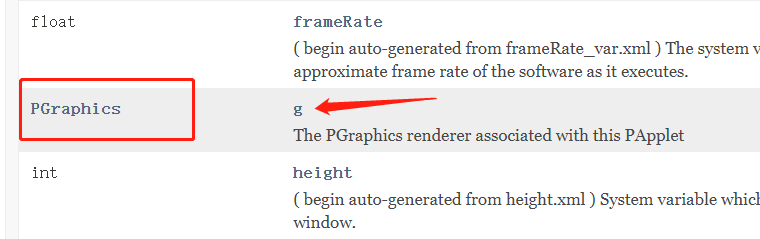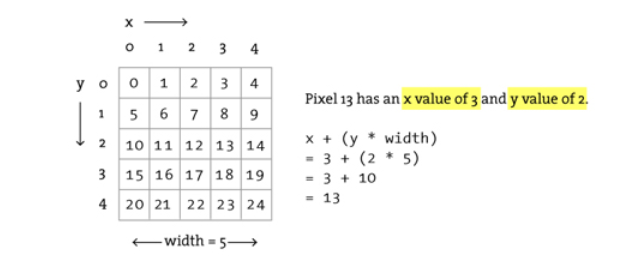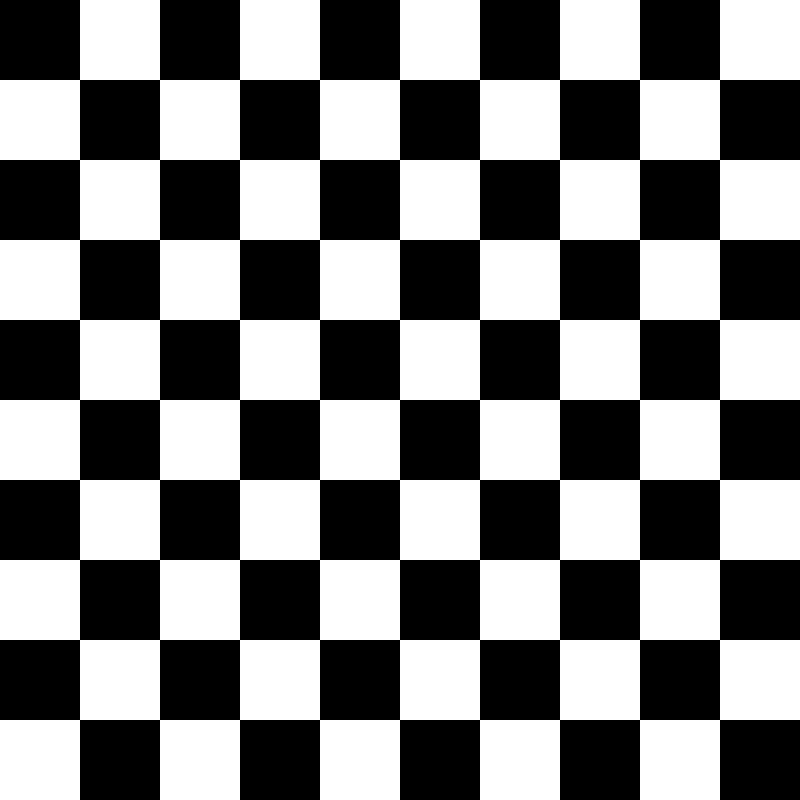# 第一步

``````void setup() {
size(400, 400);

pixels = color(0);
updatePixels();

}
````````````void setup() {
size(400, 400);  //定義的該PApplet對於surface視口大小,
//並且也創建了一個內置PGraphics，命名為g，大小是（width,height）,並做了初識化
g.pixels = color(0);
g.updatePixels();

}
``````

``````PGraphics mygraphics;
void setup() {
size(400, 400);  //定義的該PApplet對於surface視口大小
mygraphics = createGraphics(width,height);//創建graphics,大小為width，height,畫布大小
mygraphics.beginDraw();                   //這兩行實則在做PGraphics的pixels[]等屬性的初始化，
mygraphics.endDraw();                     //如果不加，下面的pixels就獲取不到，會拋空指針異常

mygraphics.pixels = color(0);
mygraphics.updatePixels();

}
``````

# 言歸正傳``````for (int x = 0; x < width; x++) {
for (int y = 0; y < height; y++) {
int loc = x + y * width;      //這是一個公式，以橫縱兩軸向進行遍歷，一位數組帶被映射到二維數組中的某個值，即圖中的某個像素點，可用loc = x + y *width來表示
pixels[loc] = color(0);     //那麼loc 這個索引號 代表的是 （x,y） 這一個像素點
}
}
``````

``````  increW = width/WCOUNT;
increH = height/HCOUNT;
``````

``````int k= 0;
void draw()
{
k ++;
if(k % 2 == 0)
pixels[x + y * width] = color(0);
if(k % 2 != 0)
pixels[x + y * width] = color(255);
}
``````

``````indexH ++ ;        //這個東東是什麼？又來了個增幅？還是開關變量？其實它才是真正控制步長的變量。
if (indexH % increH == 0)//注意表達式，同樣做求余運算，判斷是否取到0，如果是，則剛好index加了一個步長
{
indexH = 0;      //當到了一個步長，那步長計數要歸零，重新計數(其實這裡可以省略，因為求余算法可以彌補，但是為了設計算法方便，可以保留)
k ++;
}
``````

``````  increW = width/WCOUNT;
increH = height/HCOUNT;
int k = 0;
int indexH = 0;
int indexW = 0;
for (int x = 0; x  < width; x ++)
{
for (int y = 0; y < height; y ++)
{
if (k % 2 == 0)
{
pixels[x + y * width] = color(0);
} else
{
pixels[x + y * width] = color(255);
}
///////第一層邏輯控制，控制縱向繪製時每走步長數換一次K//////////
indexH ++ ;
if (indexH % increH == 0)
{
indexH = 0;
k ++;
}
///////第一層邏輯控制//////////
}
///////第二層邏輯控制，控制橫向繪製時每走步長數換一次K//////////
indexW ++ ;
if (indexW % increW == 0)
{
indexW = 0;
k ++;
}
///////第二層邏輯控制//////////

}
``````

``````int increW;
int increH;
int WCOUNT = 10;
int HCOUNT = 10;

void settings() {
size(800, 800);
}
void setup() {
increW = width/WCOUNT;
increH = height/HCOUNT;
int k = 0;
int indexH = 0;
int indexW = 0;
for (int x = 0; x  < width; x ++)
{
for (int y = 0; y < height; y ++)
{
if (k % 2 == 0)
{
pixels[x + y * width] = color(0);
} else
{
pixels[x + y * width] = color(255);
}
indexH ++ ;
if (indexH % increH == 0)
{
indexH = 0;
k ++;
}
}
indexW ++ ;
if (indexW % increW == 0)
{
indexW = 0;
k ++;
}
}
updatePixels();
}

void draw() {
}

``````

# 言外

``````int increW;
int increH;
int WCOUNT = 10;
int HCOUNT = 10;

void settings() {
size(800, 800);
}
void setup() {
increW = width/WCOUNT;
increH = height/HCOUNT;
int k = 0;
int indexH = 0;
int indexW = 0;
for (int x = 0; x  < width; x ++)
{
for (int y = 0; y < height; y ++)
{
if (k % 2 == 0)
{
set(x, y, color(0));
} else
{
set(x, y, color(255));
}
indexH ++ ;
if (indexH % increH == 0)
k ++;
}
indexW ++ ;
if (indexW % increH == 0)
k ++;
}
//updatePixels();
}

void draw() {
}

``````

# 結果